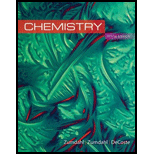# A 2.747−g sample of manganese metal is reacted with excess HCl gas to produce 3.22 L H 2 ( g ) at 373 K and 0.951 atm and a manganese chloride compound (MnCl x . What is the formula of the manganese chloride compound produced in the reaction?### Chemistry

10th Edition
Steven S. Zumdahl + 2 others
Publisher: Cengage Learning
ISBN: 9781305957404

#### Solutions

Chapter
Section### Chemistry

10th Edition
Steven S. Zumdahl + 2 others
Publisher: Cengage Learning
ISBN: 9781305957404
Chapter 5, Problem 134AE
Textbook Problem
14 views

## A 2.747−g sample of manganese metal is reacted with excess HCl gas to produce 3.22 L H2(g) at 373 K and 0.951 atm and a manganese chloride compound (MnClx. What is the formula of the manganese chloride compound produced in the reaction?

Interpretation Introduction

Interpretation: The formula of manganese chloride in the product of given reaction of manganese with HCl is needed to be identified, if the mass of manganese and volume of produced H2 are 2.747g and 3.22L respectively.

Concept introduction:

• Number of moles of a substance,

From its given mass is,

Number of moles=GivenmassMolecularmass

According to ideal gas equation,

Numberofmoles=Pressure×VolumeR×Temperature

• Balance equation of a reaction is written according to law of conservation of mass.
• Mole ratio between the reactants of a reaction is depends upon the coefficients of reactants in a balanced chemical equation.

### Explanation of Solution

Explanation

• To find: the number of moles of reacted manganese and produced H2 in the given reaction.

The number of moles of manganese is 0.05mol .

The number of moles of H2 is 0.1mol .

The mass of reacted manganese in the given reaction is given as 2.747g .

The volume of produced H2 in the given reaction is given as 3.22L .

• The number of moles of manganese.

Number of moles of a substance from its given mass is,

Number of moles=GivenmassMolecularmass

Therefore, the number of moles of manganese is,

Number of moles Mn=2.747g54.94g/mol=0.05mol

• The number of moles of H2 .

The pressure of H2 is given as 0.951atm .

The temperature is given as 373K .

According to ideal gas equation is,

Numberofmoles=Pressure×VolumeR×Temperature

Therefore, the number of moles of H2 ,

nH2=0.951atm×3.22L0.08206Latm/Kmol×373K=0.1mol

Hence, the number of moles of manganese in given reaction is 0.05mol .

The number of moles of H2 produced in given reaction is 0.1mol .

• To find: the number of moles of HCl and MnClx in the given reaction.

The number of moles of HCl reacted is 0.2mol .

The number of moles of MnClx produced is 0.05mol

• The mole ratio between reactants and products are,

The reactants of given reaction are Mn and HCl

### Still sussing out bartleby?

Check out a sample textbook solution.

See a sample solution

#### The Solution to Your Study Problems

Bartleby provides explanations to thousands of textbook problems written by our experts, many with advanced degrees!

Get Started

Find more solutions based on key concepts
How do eating disorders affect health?

Understanding Nutrition (MindTap Course List)

How is advection related to this system?

Fundamentals of Physical Geography

An important national food and nutrient intake survey, called What We Eat in America, is part of a. NHANES. b. ...

Nutrition: Concepts and Controversies - Standalone book (MindTap Course List)

What is the derivation of the word “vaccination”?

Introduction to General, Organic and Biochemistry

What is a joint?

Human Biology (MindTap Course List)

A dentists drill starts from rest. After 3.20 s of constant angular acceleration, it turns at a rate of 2.51 1...

Physics for Scientists and Engineers, Technology Update (No access codes included)

Plankton are said to be drifters, yet some of them can swim. Which is it?

Oceanography: An Invitation To Marine Science, Loose-leaf Versin